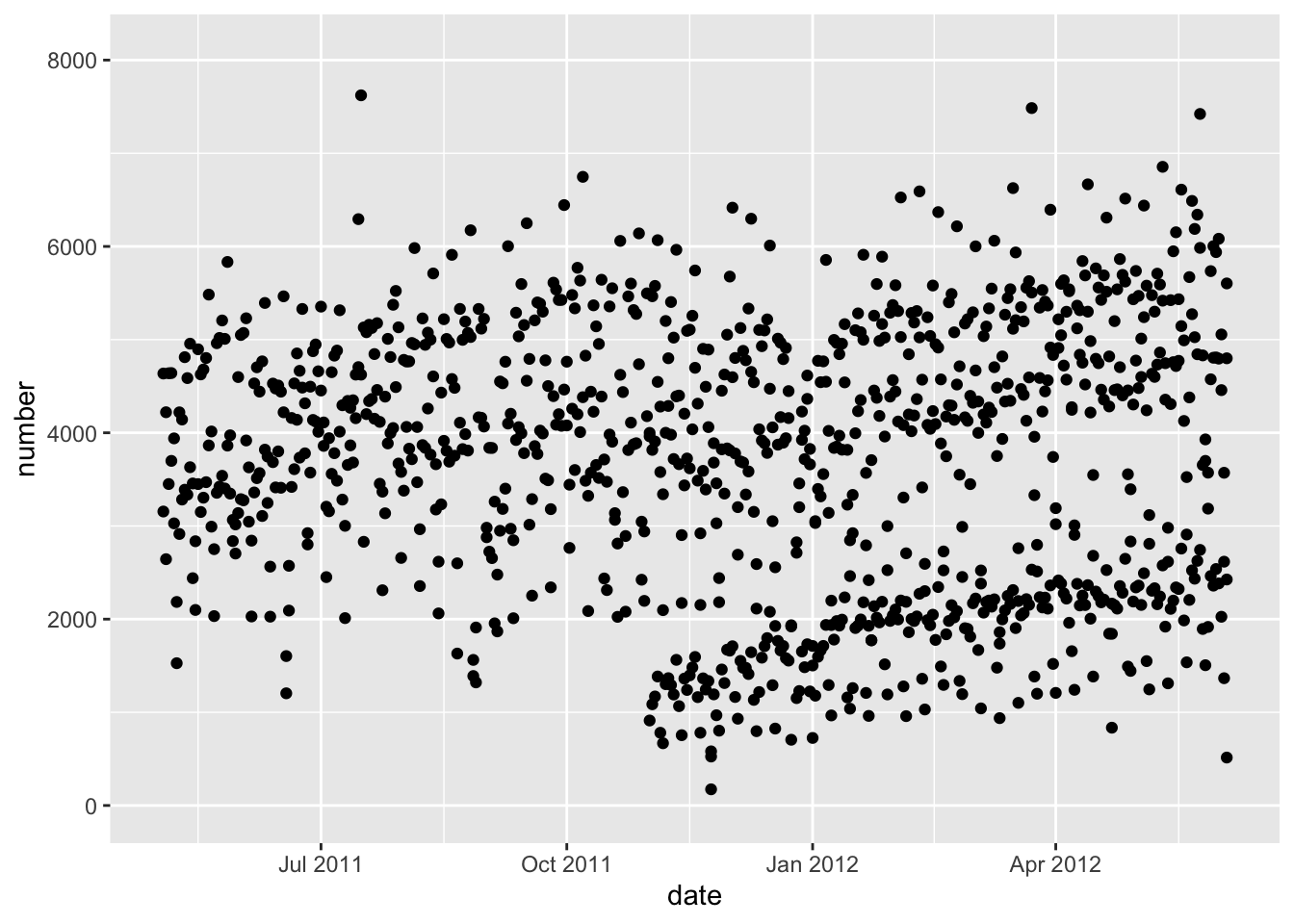``````library(readr)
library(ggplot2)
library(tidyr)
library(dplyr)
library(lubridate)
library(stringr)
library(jhur)``````

Read in the charm city circulator dataset:

`circ = read_csv("http://johnmuschelli.com/intro_to_r/data/Charm_City_Circulator_Ridership.csv")` or `circ = read_circulator()`

``circ = read_csv("http://johnmuschelli.com/intro_to_r/data/Charm_City_Circulator_Ridership.csv")``
``````## Parsed with column specification:
## cols(
##   day = col_character(),
##   date = col_character(),
##   orangeBoardings = col_double(),
##   orangeAlightings = col_double(),
##   orangeAverage = col_double(),
##   purpleBoardings = col_double(),
##   purpleAlightings = col_double(),
##   purpleAverage = col_double(),
##   greenBoardings = col_double(),
##   greenAlightings = col_double(),
##   greenAverage = col_double(),
##   bannerBoardings = col_double(),
##   bannerAlightings = col_double(),
##   bannerAverage = col_double(),
##   daily = col_double()
## )``````
``````# covert dates
circ = mutate(circ, date = mdy(date))
# change colnames for reshaping
colnames(circ) =  colnames(circ) %>%
str_replace("Board", ".Board") %>%
str_replace("Alight", ".Alight") %>%
str_replace("Average", ".Average")

# make long
long = gather(circ, "var", "number",
starts_with("orange"),
starts_with("purple"), starts_with("green"),
starts_with("banner"))
# separate
long = separate(long, var, into = c("route", "type"),
sep = "[.]")``````

or run:

``````long = read_circulator_long() %>%
rename(route = line)``````
``````## Parsed with column specification:
## cols(
##   day = col_character(),
##   date = col_character(),
##   orangeBoardings = col_double(),
##   orangeAlightings = col_double(),
##   orangeAverage = col_double(),
##   purpleBoardings = col_double(),
##   purpleAlightings = col_double(),
##   purpleAverage = col_double(),
##   greenBoardings = col_double(),
##   greenAlightings = col_double(),
##   greenAverage = col_double(),
##   bannerBoardings = col_double(),
##   bannerAlightings = col_double(),
##   bannerAverage = col_double(),
##   daily = col_double()
## )``````
``````## take just average ridership per day
avg = filter(long, type == "Average")
avg = filter(avg, !is.na(number))

# separate
type_wide = spread(long, type, value = number)
``````## # A tibble: 6 x 7
##   day    date       daily route  Alightings Average Boardings
##   <chr>  <date>     <dbl> <chr>       <dbl>   <dbl>     <dbl>
## 1 Friday 2010-01-15 1644  banner         NA      NA        NA
## 2 Friday 2010-01-15 1644  green          NA      NA        NA
## 3 Friday 2010-01-15 1644  orange       1643    1644      1645
## 4 Friday 2010-01-15 1644  purple         NA      NA        NA
## 5 Friday 2010-01-22 1394. banner         NA      NA        NA
## 6 Friday 2010-01-22 1394. green          NA      NA        NA``````

# Part 1

In these questions, try to use `ggplot2` if possible.

1. Plot average ridership (`avg` data set) by `date`.
``````q = qplot(x = date, y = number, data = avg)
q + xlim(ymd("2011/05/03", "2012/06/04"))``````
``## Warning: Removed 1871 rows containing missing values (geom_point).````````g = ggplot(avg, aes(x = date, y = number))
g + geom_point()``````